• 2020-11-20 19:25:03

1、函数FuncAnimation(fig,func,frames,init_func,interval,blit)是绘制动图的主要函数，其参数如下：

a.fig 绘制动图的画布名称

b.func自定义动画函数，即下边程序定义的函数update

c.frames动画长度，一次循环包含的帧数，在函数运行时，其值会传递给函数update(n)的形参“n”

d.init_func自定义开始帧，即传入刚定义的函数init,初始化函数

e.interval更新频率，以ms计

f.blit选择更新所有点，还是仅更新产生变化的点。应选择True，但mac用户请选择False，否则无法显

importnumpy as npimportmatplotlib.pyplot as pltfrom matplotlib.animation importFuncAnimation

#ax在第2个子图中绘制，其中行优先，

xdata, ydata=[], [] #初始化两个数组

ln,= ax.plot([], [], 'r-', animated=False) #第三个参数表示画曲线的颜色和线型，具体参见：https://blog.csdn.net/tengqingyong/article/details/78829596definit():

ax.set_xlim(0,2*np.pi) #设置x轴的范围pi代表3.14...圆周率，

ax.set_ylim(-1, 1)　　　　#设置y轴的范围returnln, #返回曲线defupdate(n):

xdata.append(n) #将每次传过来的n追加到xdata中ydata.append(np.sin(n))

ln.set_data(xdata, ydata) #重新设置曲线的值returnln,

ani= FuncAnimation(fig, update, frames=np.linspace(0, 2*np.pi, 10), #这里的frames在调用update函数是会将frames作为实参传递给“n”

init_func=init, blit=True)

plt.show()

PS:一般来说一个动图有两类函数，一类是初始化函数，另一类是需要更新的函数！！

动图绘制的路线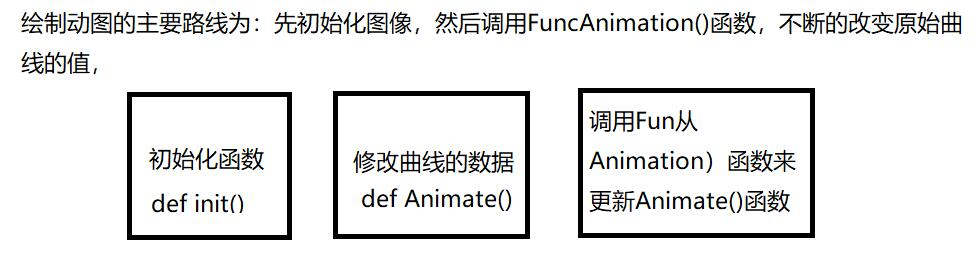更多相关内容
• 在这篇文章中，我们将熟悉并使用Matplotlib提供的animation模块，绘制动态图像。 我们将绘制一个简单的例子：在正弦函数上移动的切线。 文章目录一. FuncAnimation接口与绘图思路1. FuncAnimation接口2. 绘图思路...
• 在之前的一篇文章当中，小编当时分享了如何用Python当中的gif模块来制作gif格式的图表，厉害了，用Python绘制动态可视化图表，并保存成gif格式今天小编再给大家来介绍一种制作gif格式图表的新方法，调用的是...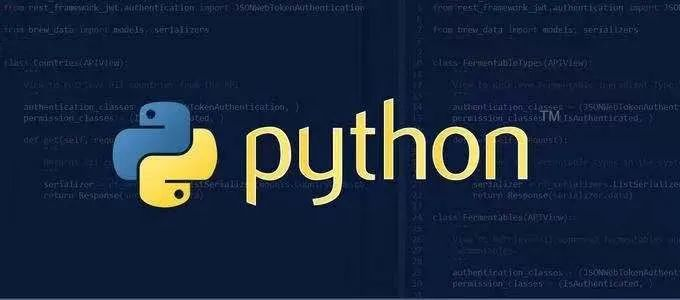在之前的一篇文章当中，小编当时分享了如何用Python当中的gif模块来制作gif格式的图表，

今天小编再给大家来介绍一种制作gif格式图表的新方法，调用的是matplotlib的相关模块，其中的步骤与方法也是相当地简单易懂。

### 下载和导入数据库

我们这次用到的数据集是bokeh模块自带的数据集，通过下面这一行代码直接就可以下载

import bokeh
bokeh.sampledata.download()

然后导入后面要用到的数据集，我们挑选的是指定国家的1950年至今不同年龄阶段的人口所占比重的数据

from bokeh.sampledata.population import data
import numpy as np

data = filter_loc('United States of America')
data.head()

output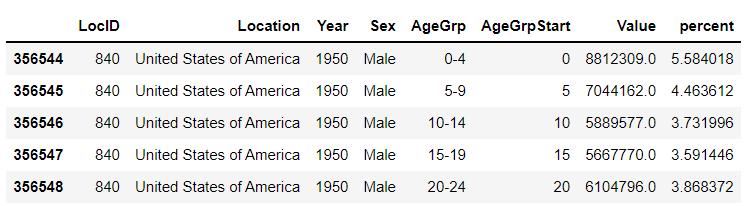### 先绘制若干张静态的图表

我们可以先绘制若干张静态的图表，然后将这几张图表合成一张gif格式的动图即可，代码如下

import seaborn as sns
import matplotlib.pyplot as plt
import matplotlib.patheffects as fx

# 绘制图表的函数
def make_plot(year):

# 根据年份来筛选出数据
df = data[data.Year == year]

# 制作图表
fig, (ax1, ax2) = plt.subplots(1, 2, sharey = True)
ax1.invert_xaxis()

ax1.barh(df[df.Sex == 'Male'].AgeGrp, df[df.Sex == 'Male'].percent, label = 'Male')
ax2.barh(df[df.Sex == 'Female'].AgeGrp, df[df.Sex == 'Female'].percent, label = 'Female', color = 'C1')

country = df.Location.iloc
if country == 'United States of America': country == 'US'

fig.suptitle(f'......')
fig.supxlabel('......')
fig.legend(bbox_to_anchor = (0.9, 0.88), loc = 'upper right')
ax1.set_ylabel('Age Groups')

return fig

我们自定义了一个绘制图表的函数，其中的参数是年份，逻辑很简单，我们是想根据年份来筛选出数据，然后根据筛选出的数据来绘制图表，每一年的图表不尽相同

years = [i for i in set(data.Year) if i < 2022]
years.sort()

for year in years:
fig = make_plot(year)
fig.savefig(f'{year}.jpeg',bbox_inches = 'tight')

output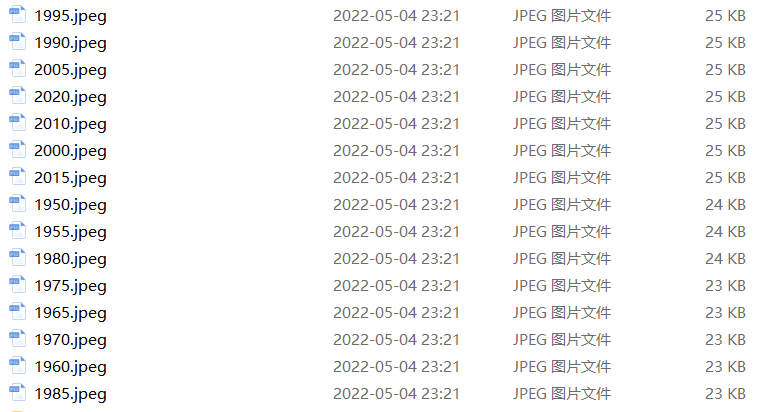这样我们就生成了若干张静态的图表，然后集合成gif格式的图表几个，代码如下

import matplotlib.animation as animation
fig, ax = plt.subplots()
ims = []

for year in years:
im = ax.imshow(plt.imread(f'{year}.jpeg'), animated = True)
ims.append([im])

ani = animation.ArtistAnimation(fig, ims, interval=600)
ani.save('us_population.gif')

output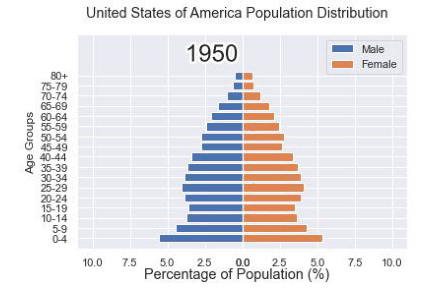### 还有另外一种思路

可能看到这儿，有人会觉得上面提到的方法稍显麻烦，毕竟我们需要先生成数十张静态的图表，要是电脑的磁盘空间有点紧张的话，或者还没有这样的一个地方来存放这数十张的图表。于是乎就会疑问道，是不是可以一步到位的来。

当然也是可以的，例如我们打算绘制1950年到2020年不同年龄阶段的人口比例分布图，首先第一步在于我们先要绘制1950年，也就是起始年，该年不同年龄阶段的人口比例分布图，代码如下

fig, (ax1, ax2) = plt.subplots(1, 2, sharey = True)

df = data[data.Year == 1955]

y_pos = [i for i in range(len(df[df.Sex == 'Male']))]
male = ax1.barh(y_pos, df[df.Sex == 'Male'].percent, label = 'Male',
tick_label = df[df.Sex == 'Male'].AgeGrp)
female = ax2.barh(y_pos, df[df.Sex == 'Female'].percent, label = 'Female',
color = 'C1', tick_label = df[df.Sex == 'Male'].AgeGrp)

ax1.invert_xaxis()
fig.suptitle('.......')
fig.supxlabel('....... (%)')
fig.legend(bbox_to_anchor = (0.9, 0.88), loc = 'upper right')
ax1.set_ylabel('Age Groups')

output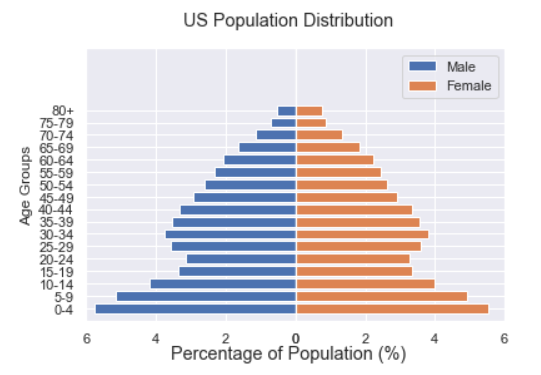然后我们自定义一个绘制图表的函数，其中参数为年份，目的在于通过年份来筛选出相对应的数据并且绘制出相对应的图表

def run(year):
# 通过年份来筛选出数据
df = data[data.Year == year]
# 针对不同地性别来绘制
total_pop = df.Value.sum()
df['percent'] = df.Value / total_pop * 100
male.remove()
y_pos = [i for i in range(len(df[df.Sex == 'Male']))]
male.patches = ax1.barh(y_pos, df[df.Sex == 'Male'].percent, label = 'Male',
color = 'C0', tick_label = df[df.Sex == 'Male'].AgeGrp)
female.remove()
female.patches = ax2.barh(y_pos, df[df.Sex == 'Female'].percent, label = 'Female',

color = 'C1', tick_label = df[df.Sex == 'Female'].AgeGrp)

text.set_text(year)
return male#, female

然后我们调用animation.FuncAnimation()方法，

ani = animation.FuncAnimation(fig, run, years, blit = True, repeat = True,
interval = 600)
ani.save('文件名.gif')

output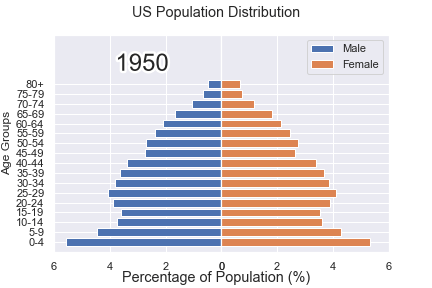这样就可以一步到位生成gif格式的图表，避免生成数十张繁多地静态图片了。

### 将若干张gif动图放置在一张大图当中

最后我们可以将若干张gif动图放置在一张大的图表当中，代码如下

import matplotlib.animation as animation

# 创建一个新的画布
fig, (ax, ax2, ax3) = plt.subplots(1, 3, figsize = (10, 3))

ims = []
for year in years:
im = ax.imshow(plt.imread(f'文件1{year}.jpeg'), animated = True)
im2 = ax2.imshow(plt.imread(f'文件2{year}.jpeg'), animated = True)
im3 = ax3.imshow(plt.imread(f'文件3{year}.jpeg'), animated = True)
ims.append([im, im2, im3])

ani = animation.ArtistAnimation(fig, ims, interval=600)
ani.save('comparison.gif')

output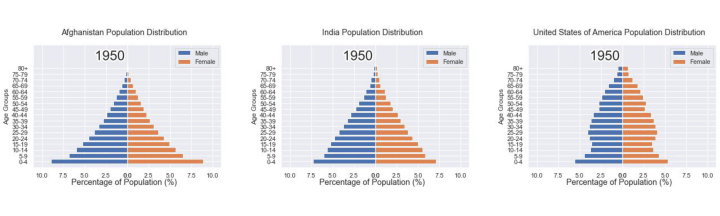推荐阅读:
入门: 最全的零基础学Python的问题  | 零基础学了8个月的Python  | 实战项目 |学Python就是这条捷径
干货:爬取豆瓣短评，电影《后来的我们》 | 38年NBA最佳球员分析 |   从万众期待到口碑扑街！唐探3令人失望  | 笑看新倚天屠龙记 | 灯谜答题王 ｜用Python做个海量小姐姐素描图 ｜碟中谍这么火，我用机器学习做个迷你推荐系统电影
趣味:弹球游戏  | 九宫格  | 漂亮的花 | 两百行Python《天天酷跑》游戏!
AI: 会做诗的机器人 | 给图片上色 | 预测收入 | 碟中谍这么火，我用机器学习做个迷你推荐系统电影
小工具: Pdf转Word，轻松搞定表格和水印！ | 一键把html网页保存为pdf！|  再见PDF提取收费！ | 用90行代码打造最强PDF转换器，word、PPT、excel、markdown、html一键转换 | 制作一款钉钉低价机票提示器！ ｜60行代码做了一个语音壁纸切换器天天看小姐姐！｜

年度爆款文案

点阅读原文，看B站我的视频！

展开全文opencv
• 这期分享Python动图制作，可视化绘制动态图，主要用于分析数据的动态变化规律，非常好使，开干。

# Python实践：画个动图玩玩，Python绘制GIF图总结

### 文章目录

上期博客《 Python实践：Pyplot绘图超简洁核心总结》，分享了Python绘图核心方法的体系化总结。

这期分享Python动图制作，可视化绘制动态图，主要用于分析数据的动态变化规律，非常好使，开干。

## 具体实现

功能需求

• 输入：不同时刻的一维数据，也即二维数据
• 过程：绘制每张图，并存入list
• 输出：一张gif图

实现思路

• 得到要绘制的不同帧横纵坐标轴数据
• 设立好图幅：坐标系、标题、标签等
• 绘制每张图，并用imageio存入list中
• 绘制完毕，将list中的每帧img，输出成gif

## Python代码

demo代码如下：

import matplotlib
matplotlib.use('Agg') # 只绘图保存，不显示图片，一定要加在import后面
import matplotlib.pyplot as plt
import numpy as np
import imageio

def generate_gif_1(data, output_path):
image_list = []
ndata = np.array(data)
row, col = ndata.shape
x = range(col)
for i in range(row):
# plot curves
# plt.figure(i)
plt.clf()  # 清除上一幅图，如果不清，则图像不断叠加 clear figure
plt.cla()  # 清除坐标轴 clear axis
plt.xlim(0, 12)   # x_min, x_max
plt.ylim(0, 1.5)  # y_min, y_max
plt.title('Val Distribu')
plt.xlabel('Time')
plt.ylabel('Val')
plt.grid(linestyle='-.')

# create gif
y = ndata[i]
plt.plot(x[:], y[:], 'b', lw=1)
plt.savefig('temp.png')
#        plt.show()
plt.pause(0.1)
plt.close()

# save gif
imageio.mimsave(output_path, image_list, 'GIF', duration=0.35)

if __name__ == "__main__":

row = 10
col = 20
data = np.random.rand(row, col) # 随机生成row行，col列，0-1的数据
output_path = './demo.gif'

generate_gif_1(data, output_path)

print('done!')



效果如下：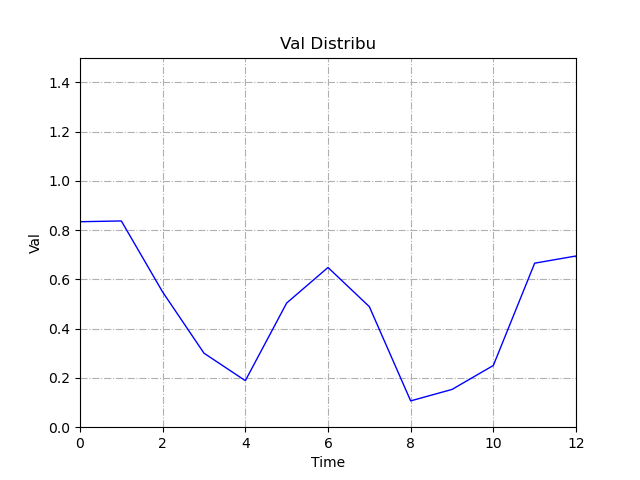## 参考资料

展开全文gif图
• 前言 想写数据动态可视化很久了，但是网上竟然没有一份能直接用的代码，...动图的核心函数是matplotlib.animation.FuncAnimation，基本用法： anim = animation.funcanimation(fig, animate, init_func=init, ...

# 前言

想写数据动态可视化很久了，但是网上竟然没有一份能直接用的代码，昨天终于狠下心来死啃了一波开发者文档搞定了这部分，贴一篇blog记录一下希望可以帮到你。

# 思路

动图的核心函数是matplotlib.animation.FuncAnimation，基本用法：

anim = animation.funcanimation(fig, animate, init_func=init, frames=100, interval=20, blit=true)
# fig: 是我们创建的画布
# animat: 是重点，是我们每个时刻要更新图形对象的函数，返回值和init_func相同
# init_func: 初始化函数，其返回值就是每次都要更新的对象，
#    告诉FuncAnimation在不同时刻要更新哪些图形对象
# frames: 相当于时刻t，要模拟多少帧图画，不同时刻的t相当于animat的参数
# interval: 刷新频率，毫秒
# blit: blit是一个非常重要的关键字，它告诉动画只重绘修改的部分，结合上面保存的时间，
#    blit=true会使动画显示得会非常非常快


动图绘制的关键是动态更新数据并刷新图像，更新数据需要写一个animat函数，看具体实现：

# 实现

给出代码及对应注释，你应该会秒懂：

# -*-coding:utf-8-*-
import random
from matplotlib.backends.backend_agg import FigureCanvas
from matplotlib import pyplot as plt
from matplotlib import animation
import numpy as np
import seaborn as sns

gesture_i =  * 2200
gesture_q =  * 2200
acc_first =  * 6
acc_second =  * 6

acc_first_max_index = 0
acc_second_max_index = 0
acc_first_max = 0
acc_second_max = 0

cur_data_count = 0

update_first_flag = False
update_second_flag = False
name_list = ["Static", "Approach", "Apart", "Click", "Flip", "Circle"]

# 创建画布，包含4个子图
fig = plt.figure(figsize=(15, 10))

fig.figimage(bgimg,resize=True)#设置窗口自适应（背景图片）

ax1.set_facecolor('none')#设置该子图背景透明，其他子图同理

ax2.set_facecolor('none')

ax3.set_facecolor('none')

ax4.set_facecolor('none')

# 绘制初始图形
bar1 = ax3.bar(range(len(acc_first)), acc_first, color='rgb', tick_label=name_list)

bar2 = ax4.bar(range(len(acc_first)), acc_first, color='rgb', tick_label=name_list)

x = np.arange(0, 2200, 1)  # x轴

ax1.set_ylim(-1, 1)#设置y轴范围为-1到1
line1, = ax1.plot(x, gesture_i,color='coral')

ax2.set_ylim(-1, 1)
line2, = ax2.plot(x, gesture_q,color='coral')

#初始化函数
def init():
# 构造开始帧函数init
# 改变y轴数据，x轴不需要改
line1.set_ydata(gesture_i)
line2.set_ydata(gesture_q)
bar1 = ax3.bar(range(len(acc_first)), acc_first, color='rgb', tick_label=name_list)
bar2 = ax4.bar(range(len(acc_second)), acc_second, color='rgb', tick_label=name_list)

ax1.set_xlabel("I")
ax2.set_xlabel("Q")

return line1, line2, ax1  # 注意返回值，我们要更新的就是这些数据

#更新图像的函数
def animate(i):
#注意这里必须要用global声明，不然可能出现无法动态更新数据的情况
global gesture_i
global gesture_q
global update_first_flag
global update_second_flag

line1.set_ydata(gesture_i)

ax3.cla()
bar1 = ax3.bar(range(len(acc_first)), acc_first, color='rgb', tick_label=name_list)
ax3.legend()

ax4.cla()
bar2 = ax4.bar(range(len(acc_second)), acc_second, color='rgb', tick_label=name_list)
ax4.legend

return line1, line2, ax1

def draw_view():
# 调用FuncAnimation函数生成动画。参数说明：
# fig 进行动画绘制的figure
# func 自定义动画函数，即传入刚定义的函数animate
# frames 动画长度，一次循环包含的帧数
# init_func 自定义开始帧，即传入刚定义的函数init
# interval 更新频率，以ms计
# blit 选择更新所有点，还是仅更新产生变化的点。应选择True，但mac用户请选择False，否则无法显示动画
ani = animation.FuncAnimation(fig=fig,
func=animate,
frames=100,
init_func=init,
interval=100,
blit=False)
plt.show()

if __name__ == '__main__':
draw_view()


说明：实现动态数据可视化的思路是将绘制图像所用的数据写成全局变量，然后动态更新你的数据，UI层会一帧一帧地刷新图像，这样只要你的数据在变，图像就会是变化的，给一张效果图：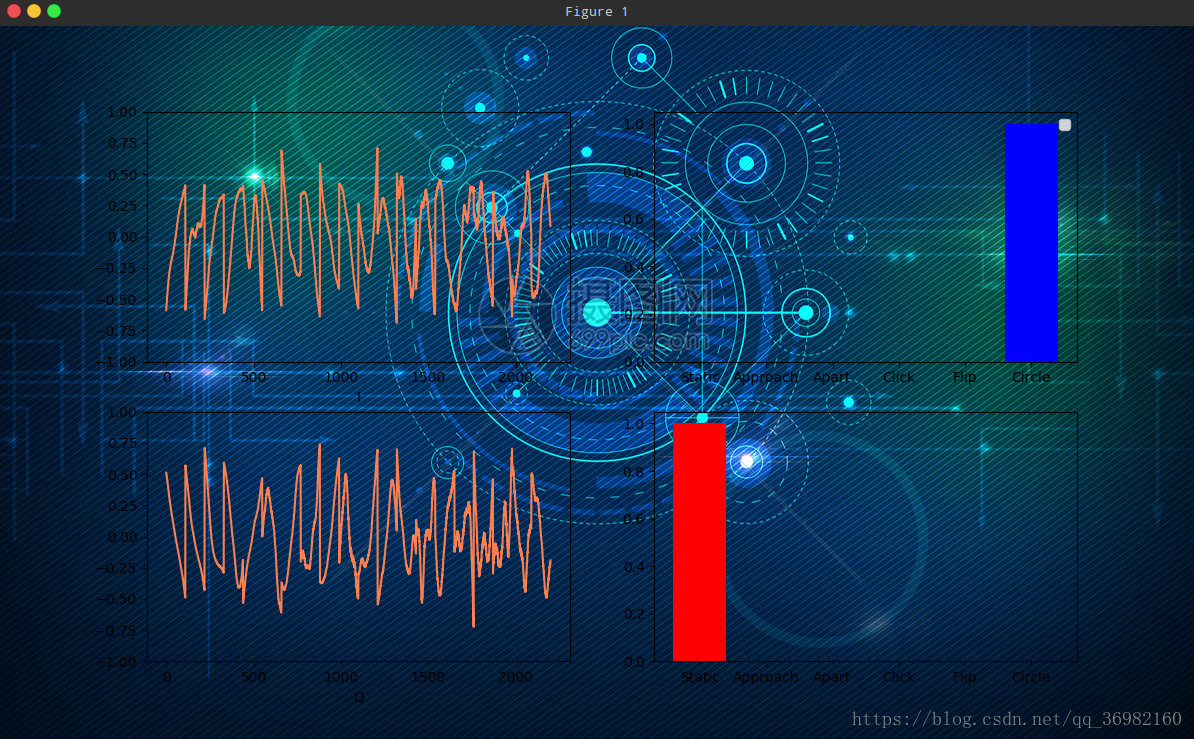展开全文matplotlib
• python绘制北京市公交线路动态图，包括获取百度秘钥、整理公交车地理数据以及可视化绘制
• 今天小编给大家介绍一款可视化模块，使用它可以绘制出十分惊艳的动图效果，那么当然第一步我们首先是要安装一下该模块，通过pip命令行来安装pipinstallipyvizzu牛刀小试我们首先来简单地使用该模块来绘制一张动图，...
• 今天小编给大家介绍一款可视化模块，使用它可以绘制出十分惊艳的动图效果，那么当然第一步我们首先是要安装一下该模块，通过pip命令行来安装pipinstallipyvizzu牛刀小试我们首先来简单地使用该模块来绘制一张动图，...人工智能 机器学习
• 主要介绍了Python 绘制可视化折线图的方法，文中讲解非常细致，代码帮助大家更好的理解和学习，感兴趣的朋友可以了解下
• 主要介绍了python如何绘制疫情图，帮助大家理解和学习用python绘制图像，感兴趣的朋友可以了解下
• 通常我们用 Python 绘制的都是二维平面图，但有时也需要绘制三维场景图，比如像下面这样的： 这些图怎么做出来呢？今天就来分享下如何一步步绘制出三维矢量（SVG）图。 八面体 我们先以下面这个八面体为例。 1 ...
• 主要介绍了python之matplotlib学习绘制动态更新图实例代码，文中涉及具体实现代码，演示效果及运行时出现的问题分析等相关内容，小编觉得还是挺不错的，这里分享给大家，需要的朋友可以参考下
• 这篇文章利用的是matplotlib.pyplot.plot的工具来绘制折线图，这里先给出一个段代码和结果图： # -*- coding: UTF-8 -*- import numpy as np import matplotlib as mpl import matplotlib.pyplot as plt #这里导入...
• 主要介绍了基于Python绘制个人足迹地图,文中通过示例代码介绍的非常详细，对大家的学习或者工作具有一定的参考学习价值,需要的朋友可以参考下
• 原标题：如何使用 Python 绘制一套动态图形？来源：机器之心在读技术博客的过程中，我们会发现那些能够把知识、成果讲透的博主很多都会做动态图表。他们的图是怎么做的？难度大吗？这篇文章就介绍了 Python 中一种...
• ## 使用Python制作GIF动图

万次阅读 多人点赞 2019-03-31 13:51:57
最近偶尔看到一位朋友的公众号中提到了使用Python生成GIF动图。看着挺有趣的，于是尝试一下。 其实是有很多制作动图的软件，但是自己丰衣足食，使用代码来实现，想想还是挺不错的。 效果图 那我自己的图为例，首先来...
• 通常大家做出来的图表，绝大部分都是静态的，有时会显得不够吸引人。今天小F就给大家介绍一下，如何用Python绘制动态图表。主要是使用到Matplotlib+imageio，其中Matplo...列表
• python 如何绘制动图，举个例子：将300张SST月均数据绘制生成gif动画 最近，在绘制SST月均数据时，突然想看看近几十年sst的大致变化过程。说白了就是绘制一张gif动画，也算是学习过程啦。 大概思路就是: 首先生成你...
• import matplotlib.pyplot as plt import numpy as np x = np.linspace(-1,1,10000) fig = plt.figure() ax = fig.add_subplot(111) ax.set_aspect('equal') P = 5 for p in np.arange(0.3,P,0.2): ...
• # Python使用matplotlib绘制动态正弦曲线图 保存为gif动图 1. 使用matplotlib绘制正弦曲线； 2. 逐帧前进形成动画，可在界面显示也可保存为gif动画。
• 分别基于Python Pillow库和Imageio库，实现批量读取图片绘制GIF动图
• 本文通过Python演示了矢量控制中的坐标变换过程，需要读者熟悉矢量控制中的坐标变换理论。坐标变换
• ## python matplotlib绘制gif动图以及保存

万次阅读 多人点赞 2019-01-04 15:22:39
python matplotlib绘制gif动图以及保存 标签： python matplotlib 谨以此文纪念我两天来的悲剧 昨天我用lstm拟合sin曲线，看到别人画的做的动图很好看，并且还能保存下来，所以我也想做着玩一下，但是没想到在网上...
• 先来看看绘制的动态水球图：没有安装PyEcharts的，先安装PyEcharts：# 安装pyecharts模块，直接安装就是最新的版本pip install pyecharts安装好PyEcharts之后，就可以将需要使用的模块进行导入：from pyecharts ......